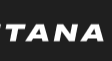# Three Number Sum in Python

I recently created a solution for the three number sum problem in JavaScript, and now that I want to practice my Python, I thought I’d create a similar solution in Python. Let’s begin.

# The Prompt

Create a function that takes an array and a target integer that represents the sum. The function should find all triplets in the array that sum up to the target integer.

The numbers should be in ascending order.

If on three numbers sum up to the target integer, then the function should return an empty array.

# The Code

First, we’ll be sorting the array and create a variable to represent the final array.

Now, we’ll initiate a for loop to traverse the input array. At the same time, we’l create a left and right variable(s) that will act as pointers.

Now, while the left value is less than the right value, create a variable that will act as the current sum, this will be a comparison to the target sum

If the current sum is equal to the target sum, then we’ll need to append the value to the array we created.

If the value was less than the target, then we’ll have to increment the left value.

If the value was greater than the target, then decrement the right value.

Finally, return the array representing the triplets.

The final code should look like the following:

# Complexity Analysis

The time complexity of this solution is O(n ^ 2) time, where (n) represents the length of the input array.

The space complexity of this solution is O(n) space, since we’re created an array to store our final values.

--

--

--

## More from Karan S. Chauhan

Love podcasts or audiobooks? Learn on the go with our new app.

## Steganography — Hiding Files An Inside Image## How to install Anbox on Kali Linux 2022.1## Creating a Local test environment: Installing CentOs to VirtualBox & Troubleshooting## An Intuitive Understanding on Tensor Sum Dimension with Pytorch## Kattana — the benefits of the project.## AWS Certified Solutions Architect Exam Preparation Guide## Flutter vs React Native## AMA coming soon on Infinity Chain Community!## My journey from the world of Java and Python to C++## Everything is object in Python